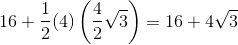## Example Questions

### Example Question #617 : Act Math

A certain rectangle is 4 times as long as it is wide. Suppose the length and the width of this rectangle are both halved. The area of the first rectangle is how many times as large than the area of the second rectangle?Explanation:

This question actually has a lot of extraneous information that you won't need to solve the problem. With any rectangle, when the dimensions are halved, the area of the resulting rectangle will always beof the original.

Another way to solve this is by calculating the area of the first rectangle. In this case we let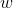be the width and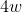be the length.

Therefore the area becomes: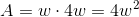For the second rectangle our width becomes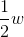and length becomes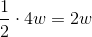Thus the area of this rectangle is: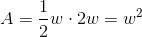Therefore rectangle one has an area 4 times larger than that of rectangle two.

### Example Question #612 : Act Math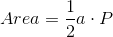A gardener is tending to a garden in the shape of a regular octagon. Its apothem isft. What is the area of the garden?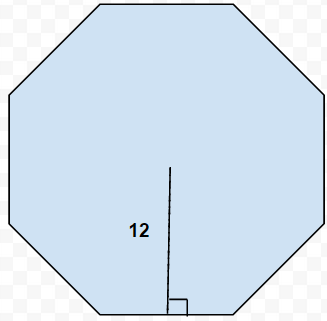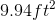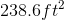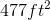Not enough information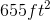Explanation:

In this type of problem, the first step is to determine what information are you missing that prevents you from solving the problem as it is? Considering that the formula to find the area of a regular polygon is, where a is apothem and P is perimeter, it can be deduced that no information is provided about the length of each side of the octagon. However, enough information is provided for that piece of information to be calculated through the use of right triangles.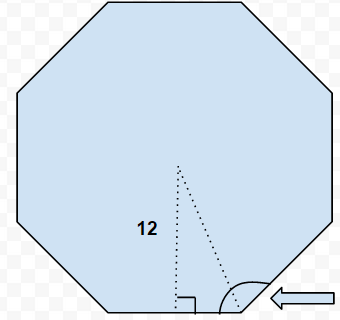In order to proceed, the internal angles of a regular octagon must be caluclated. The arrow points to an internal angle. This can be solved using the equation: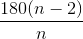, where n is the number of sides. The internal angle is calculated to be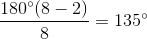. When creating the right triangle, as shown in the previous figure, the internal angle is bisected. As a result, the triangle may be seen as:

.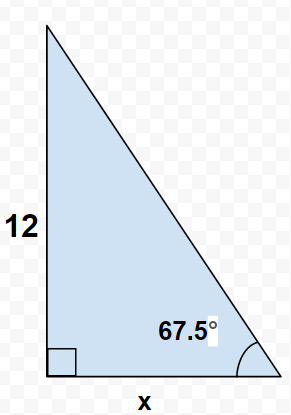Now, using trigonometric functions, the base of the triangle can be calculated to be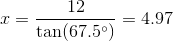. Because the base of the triangle is only half the total length of one of the sides of the octagon, this number has to be multiplied by 2.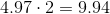9.94 will be used to calculate the octagon's perimeter: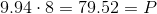Now all that's left is to substitute in the numbers obtained for apothem and perimeter to determine the area: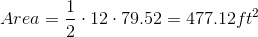### Example Question #619 : Act Math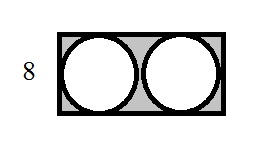Two circles are inscribed on the inside of a rectangle and are side-by-side. What is the area of the shaded region?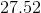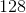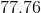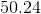Explanation:

When attempting to solve the area for the space between two shapes, find the area of both shapes and subtract the area of the smaller shape (in this case, the circles) from the larger shape (the rectangle).

To do this, we must first figure out the area of the circles given.When a circle is inscribed on the inside of a square or rectangle (in other words, it will touch all sides of the shape, the diameter of the circle will be the same as the square. In this case, since the shape is a rectangle, the width is equal to the diameter of one circle and the length is equal to the diameter of both circles.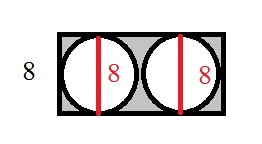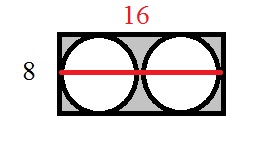Use this to solve for the area of the cirlces: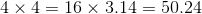Each circle has an area of 50.24 square units, so the total area of the circles is 100.48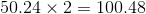Then, solve for the area of the rectangle: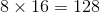Then subtract the two in order to find the area of the shaded space.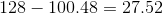### Example Question #620 : Act Math

Third grader Sammy drew a house by drawing a square with an equilateral triangle on top. The bottom of the square is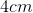long. Sammy would like to know the area of the house in order to procure the appropriately sized crayon to color it in.

Which of the following is nearest to the area of the house in square centimeters?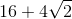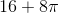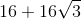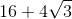Explanation:

The area of the house is comprised of the area of the square base and the triangle roof. The square has side length, so the area of the square is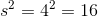and the area of the equilateral triangle roof is given by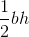, whereis the base of the triangle, andis the height.

The base of the triangle sits on top of the square, and therefore is the same length,.

The height of an equilateral triangle is given by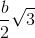.

This can be found by dividing the equilateral triangle into two right triangles (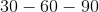right triangles to be more specific).

All together, the area of the square and triangle is